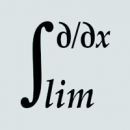# MathematicsCalculus of finite differences without variables This article contains an elementary introduction to calculus of finite differences. Instead of working with a variable, say $n$, it uses a (...) May 8, 2014 – Jakub Marian – MathematicsDefinite integration without variables In the previous article, we discussed how to integrate functions without using variables, but only in the case of indefinite integration. (...) April 23, 2014 – Jakub Marian – MathematicsQuantification without variables I have written several articles about a way to do classical calculus without using variables, e.g. how to differentiate or integrate (...) February 15, 2014 – Jakub Marian – MathematicsSecond substitution method for integration without variables This article is based on my article about integration without variables which you may want to read first in order to understand what’s (...) February 1, 2014 – Jakub Marian – MathematicsIndefinite integration without variables In this article, I’ll assume you have already read my article on differentiation without variables. Just as a quick reminder: $\iota$ (...) January 12, 2014 – Jakub Marian – Mathematics

## Subscribe to my educational newsletter

to receive a weekly summary of new articlesIntegral of $\cos^2(x)$ Let’s use integration by parts: If we apply integration by parts to the rightmost expression again, we will get $∫\cos^2(x)dx = ∫\cos^2(x)dx$, which is not very useful. The trick is to rewrite the $\sin^2(x)$ in the second step as $1-\cos^2(x)$. Then we get (...) November 24, 2013 – Jakub Marian – MathematicsRender LaTeX in Wikipedia using Mathjax Let’s face it—mathematical expressions rendered as images look ugly, especially in inline formulas. Also, if you, like me, have all (...) October 26, 2013 – Jakub Marian – Blog, MathematicsDifferentiation (derivatives) without variables To be able to differentiate a function without using variables, we have to be able to denote the function we want to differentiate without (...) October 14, 2013 – Jakub Marian – MathematicsHow to define functions without using variables First, note that this article is not about the definition of elementary functions, such as $\sin$ and $\cos$, and operations, like $a^b$, (...) October 11, 2013 – Jakub Marian – MathematicsApplying an operator with respect to a variable There are seemingly two kinds of “operations” used in mathematics when it comes to working with functions; you can either take a function (...) October 10, 2013 – Jakub Marian – Mathematics